시간 제한 메모리 제한 제출 정답 맞은 사람 정답 비율
2 초 512 MB 4 4 3 100.000%

## 문제

You are designing a virtual machine for a new programming language called Lombok. The Lombok Virtual Machine (LVM) runs an assembler-like machine code. It operates on a stack and a single register.

In detail, the instructions work as follows:

PUSH x: This instruction pushes a given integer onto the stack. If the stack for example looks like this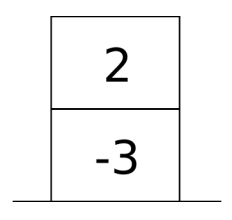and the machine executes the instruction PUSH -11, the stack looks like this afterwards: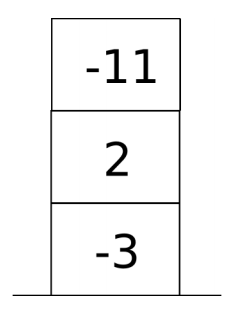STORE: This instruction takes the topmost integer from the stack and stores it in the register. If the stack for example looks like this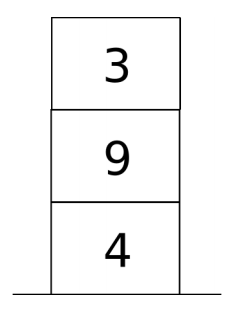the register contains any integer, and the machine executes the instruction STORE, the stack looks like this afterwards: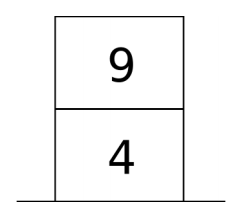and the register contains the integer 3.

LOAD: This instruction copies the content of the register and pushes it onto the stack. If the register for example contains the integer 6, the stack looks like this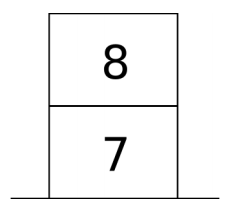and the machine executes the instruction LOAD, the stack looks like this afterwards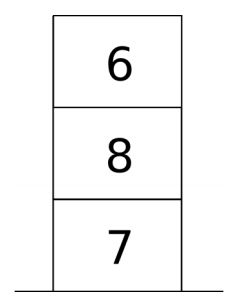and the register still contains the integer 6.

PLUS: This instruction takes the two topmost integers from the stack, adds them, and pushes the resulting integer back onto the stack. If the stack for example looks like this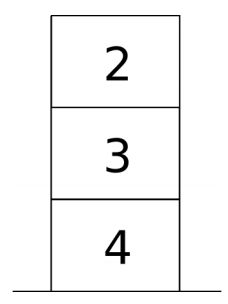and the machine executes the instruction PLUS, the stack looks like this afterwards: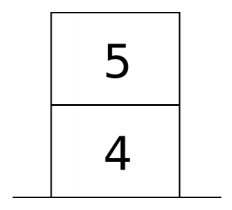TIMES: This instruction takes the two topmost integers from the stack, multiplies them, and pushes the resulting integer back onto the stack. If the stack for example looks like this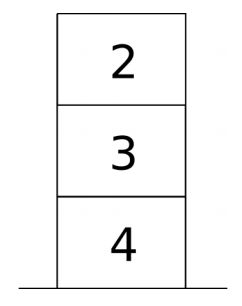and the machine executes the instruction TIMES, the stack looks like this afterwards: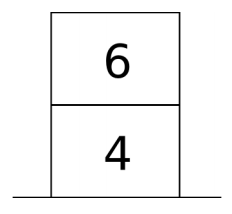IFZERO n: This instruction removes the topmost integer from the stack, and checks if it is equal to 0. If that is the case, it jumps to the nth instruction (start counting at 0). If not, the machine continues as usual with the next instruction. See example below.

DONE: Finally, the DONE instruction prints out the integer on top of the stack, and terminates the program regardless of the following instructions.

Computation starts with the first instruction. Initially, the stack is empty and the register contains the number 0.

## 입력

The first line of the input contains an integer n between 2 and 1000, indicating the number of instructions of the program. The following n lines contain the program instructions. Arguments are separated by a space character from the preceding instruction.

## 출력

The output consists of a single integer, which is the result of the first DONE instruction encountered during execution.

## 예제 입력 1

14
PUSH 5
STORE
PUSH -1
PLUS
STORE
IFZERO 13
TIMES
PUSH 0
IFZERO 3
DONE


## 예제 출력 1

120


## 힌트

0: PUSH 5
1: STORE
4: PUSH -1
5: PLUS
6: STORE
8: IFZERO 13
10: TIMES
11: PUSH 0
12: IFZERO 3
13: DONE

Note that the line numbers are added for illustration; they are not part of the program. After executing the first 8 instructions, the stack looks like this: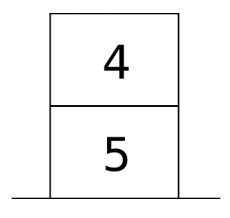and the register contains the integer 4. The next instruction IFZERO 12 removes the integer 4 from the stack. Since 4 is not equal to 0, the instructions LOAD, TIMES and PUSH 0 are executed next, resulting in the stack: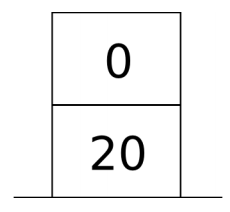The next instruction IFZERO 3 removes 0 from the stack and jumps to second LOAD instruction (address 3), since 0 is equal to 0. Eventually, this program computes 5 × 4 × 3 × 2 × 1 and prints out the resulting integer 120.

You can assume that the given program is correct; there will be no infinite loops, and the program will never attempt to remove an integer from an empty stack. You can assume that the stack does not grow bigger than 100, that the argument of PUSH is an integer not smaller than −10000 and not larger than 10000, and that the argument of ISZERO is an integer not smaller than 0 and not larger than 1000.